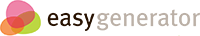# Uniform Motion Terms and Symbols

## Uniform Motion terms

### Terms and definition matching review

Now that you've seen the terms and definitions, see if you can match them up correctly.
• Scalar quantity
A quantity specified only by its magnitude, does not have direction
• Vector quantity
A quantity specified by magnitude and direction
• Position
Where you are; How far from some zero point and in which direction
When or at what instantaneous time
• Time interval
Elapsed time between two clock readings
• Distance
Length of path traveled during a time interval
• Displacement
How far you are from where you started and in which direction
• Average velocity
Average speed and direction over a time interval; the slope of a position vs. time graph
• Velocity
How fast something is moving and in which direction
• Speed
How fast something is moving; does not take direction into account

## Uniform Motion symbols

### What is the symbol for position?

•••### What is the symbol for clock reading?

•••### What is the symbol for time interval?

•••### How would you write the symbol for distance?

• The symbol for distance is delta x (a triangle in front of an x).
• The symbol for distance is delta x (a triangle in front of an x) with an arrow over the x indicating it has direction.

### How would you write the symbol for displacement?

• The symbol for displacement is delta x (a triangle in front of an x).
• The symbol for displacement is delta x (a triangle in front of an x) with an arrow over the x indicating it has direction.

### This is the symbol for average velocity.

•••### This is the symbol for velocity.

•••### This is the symbol for speed.

•••